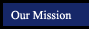Section Title Notebook files More examples (in pop-up window) 7.1 Multiplying Monomials new per4 per6 notebook express 7.2 Dividing Monomials new per4 per6 notebook express 7.3 Polynomials new per4 per6 notebook express 7.4 Adding and Subtracting Polynomials new per4 per6 notebook express 7.5 Multiplying a Polynomial by a Monomial new per4 per6 notebook express 7.6 Multiplying Polynomials new per4 per6 notebook express

Online Scientific Calculator from Calculator.com

Math Glossary
(more sophisticated than it looks!)